• Размер: 341.5 Кб
• Количество слайдов: 16

## Описание презентации Magnetic Phenomena Magnetic Field Magnetic Field по слайдам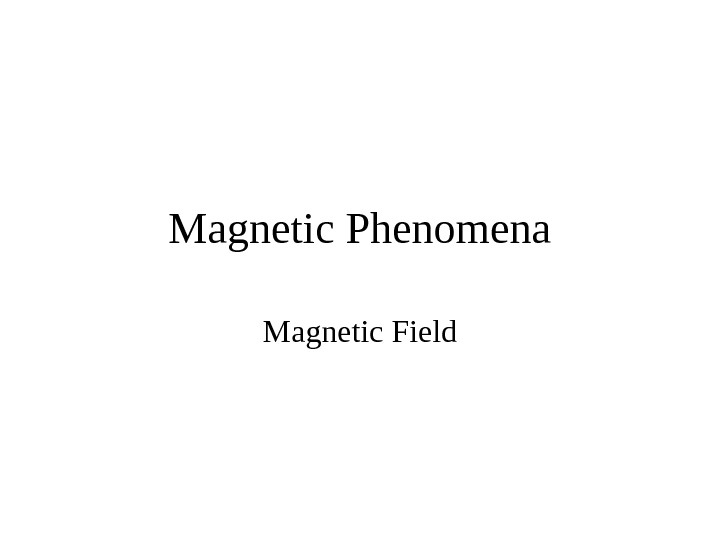Magnetic Phenomena Magnetic Field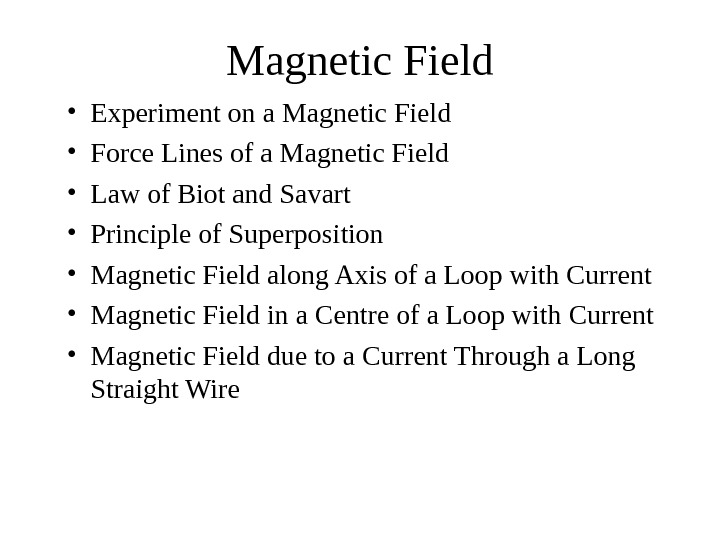Magnetic Field • Experiment on a Magnetic Field • Force Lines of a Magnetic Field • Law of Biot and Savart • Principle of Superposition • Magnetic Field along Axis of a Loop with Current • Magnetic Field in a Centre of a Loop with Current • Magnetic Field due to a Current Through a Long Straight Wire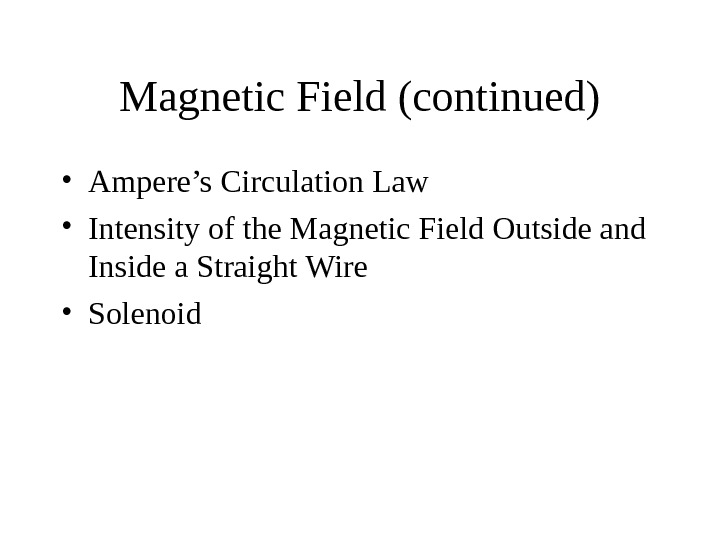Magnetic Field (continued) • Ampere’s Circulation Law • Intensity of the Magnetic Field Outside and Inside a Straight Wire • Solenoid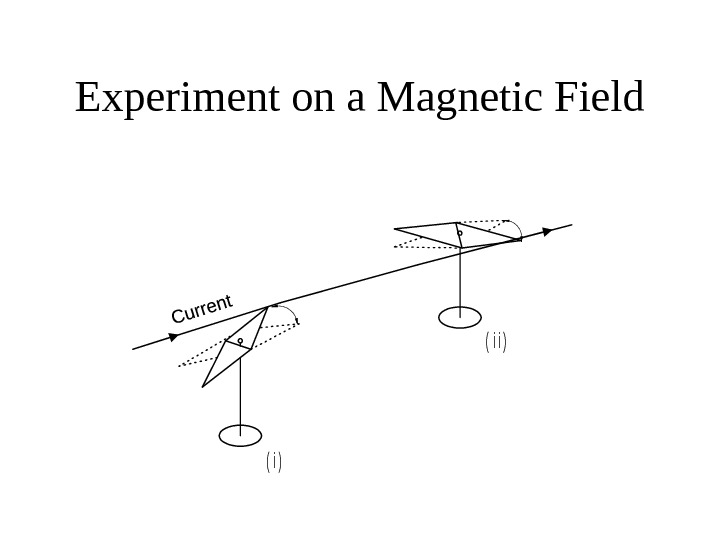Experiment on a Magnetic Field( i ) ( i i )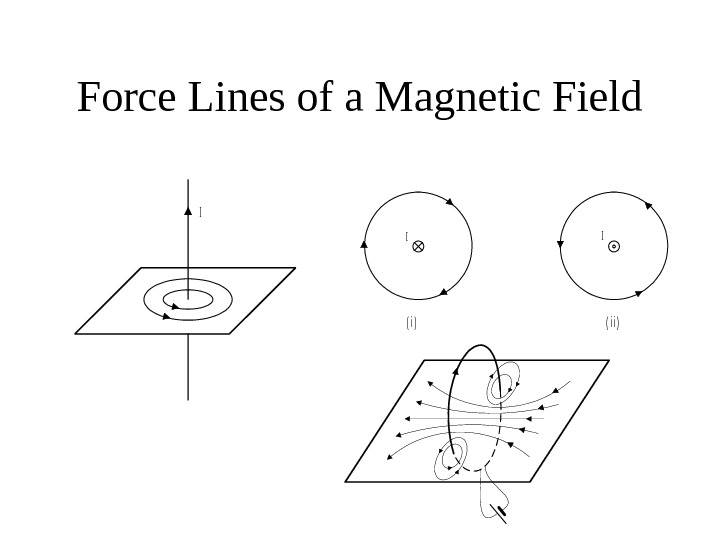Force Lines of a Magnetic Field. I I ( i )( i i ) I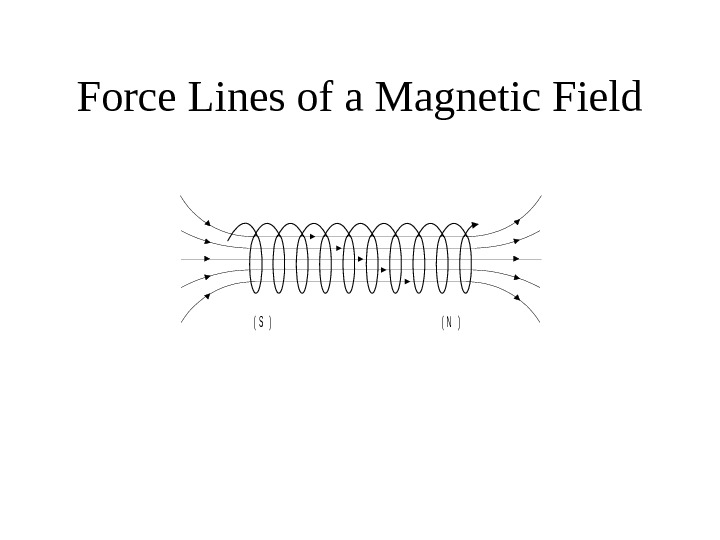Force Lines of a Magnetic Field( S )( N )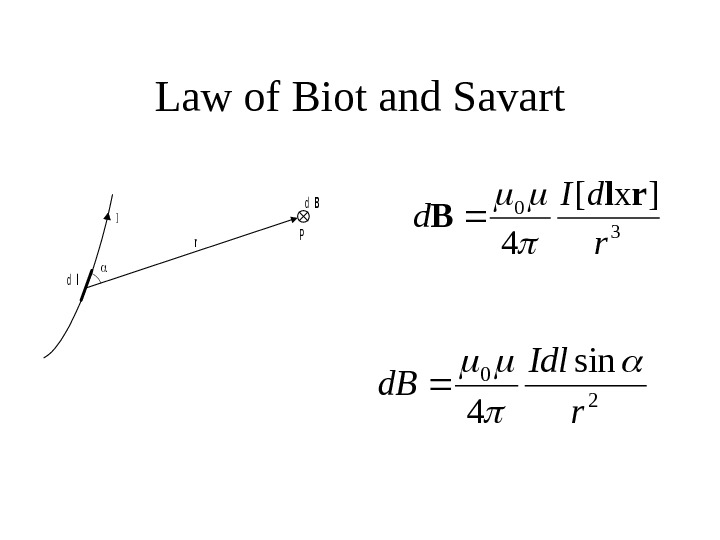Law of Biot and Savartdl I r. P d. B 3 0]x[ 4 r d. I d rl B 2 0 sin 4 r Idl d.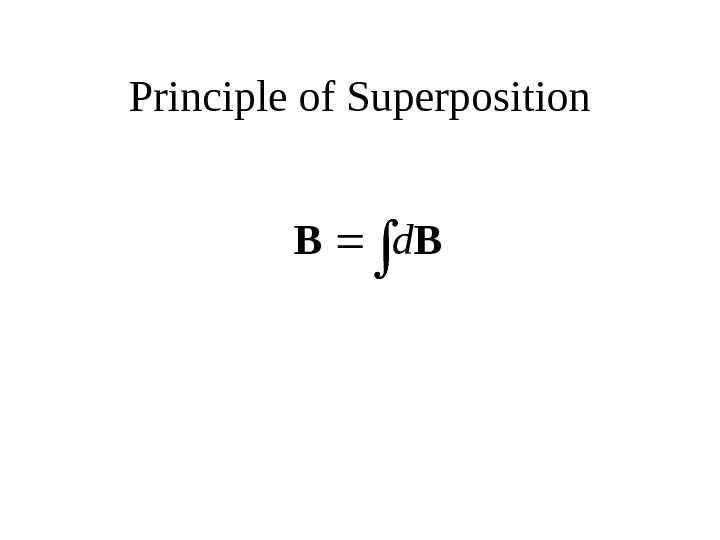Principle of Superposition BB d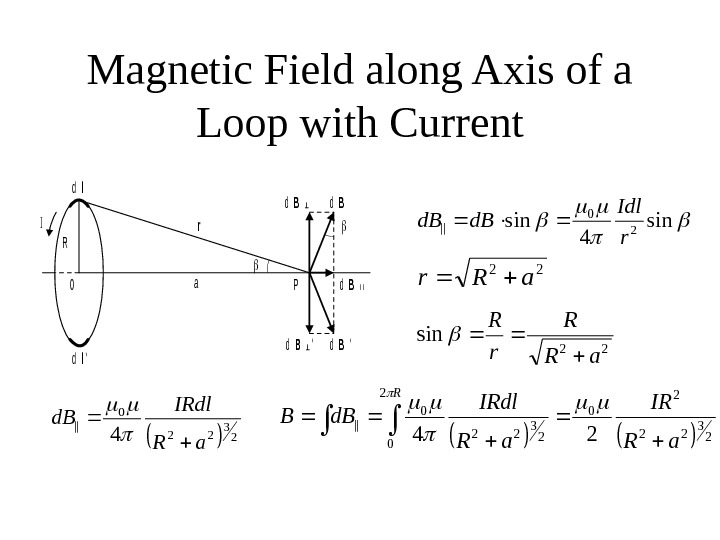Magnetic Field along Axis of a Loop with Current. I R 0 a r dl dl’ d. B’ d. Bl l d. B’ P sin 4 sin 20 || r Idl d. B 22 a. Rr 22 sin a. R R r R 23 220 || 4 a. R IRdl d. B 23 22 2 02 0 23 220 ||24 a. R IRdl d. BB R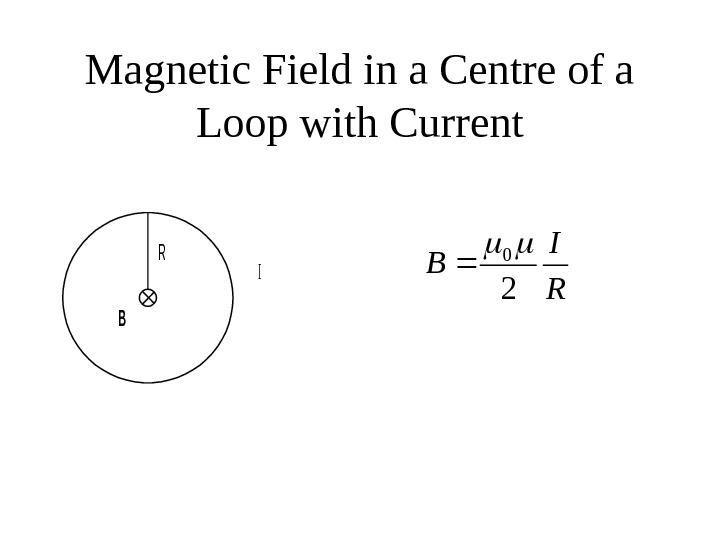Magnetic Field in a Centre of a Loop with Current. B R I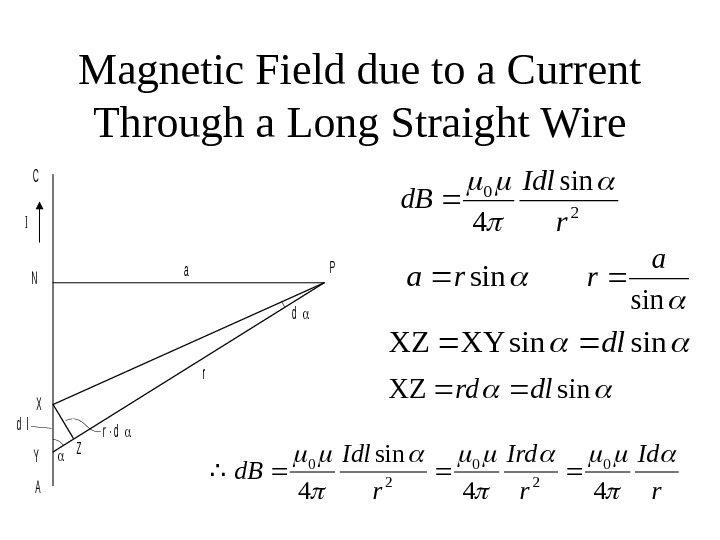Magnetic Field due to a Current Through a Long Straight Wire. I C a. P r X Y A Z d lr ∙ d d N 2 0 sin 4 r Idl d. B sinra sin a r sinsin. XYXZdl sin. XZdlrd r Ird r Idl d. B 44 sin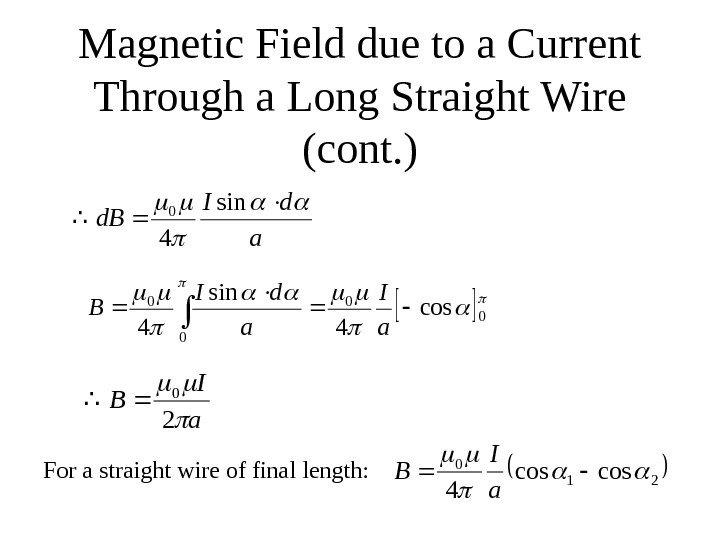Magnetic Field due to a Current Through a Long Straight Wire (cont. )a d. I d. B sin 4 0 0 0 cos 4 sin 4 a I a d. I B a I B 2 0 For a straight wire of final length: 21 0 coscos 4 a I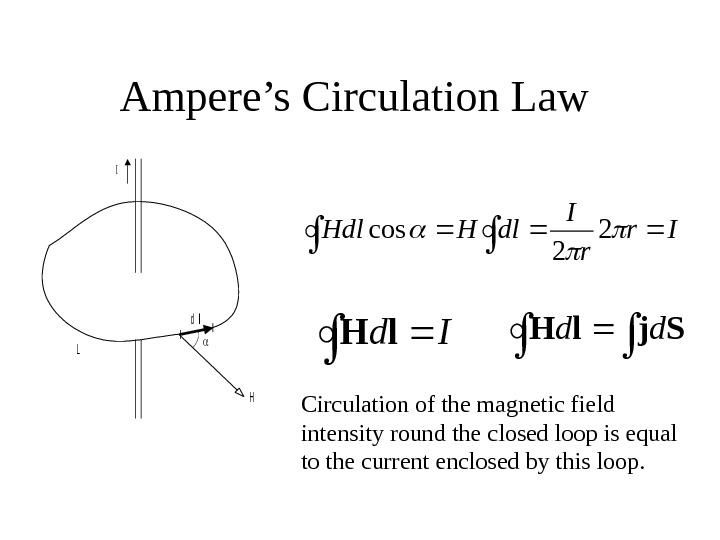Ampere’s Circulation Law I L H dl Ir r I dl. HHdl 2 2 cos Circulation of the magnetic field intensity round the closed loop is equal to the current enclosed by this loop. Id l. H Sjl. H dd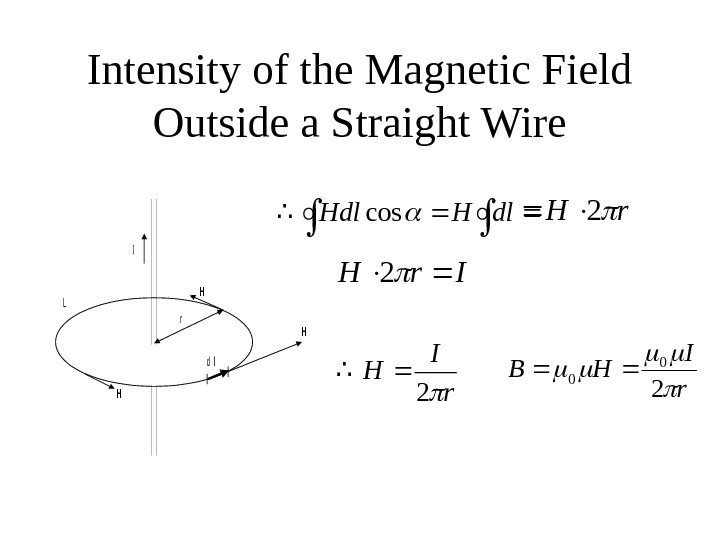Intensity of the Magnetic Field Outside a Straight Wire. H H L dl H r I dl. HHdlcosr. H 2 Ir. H 2 r I H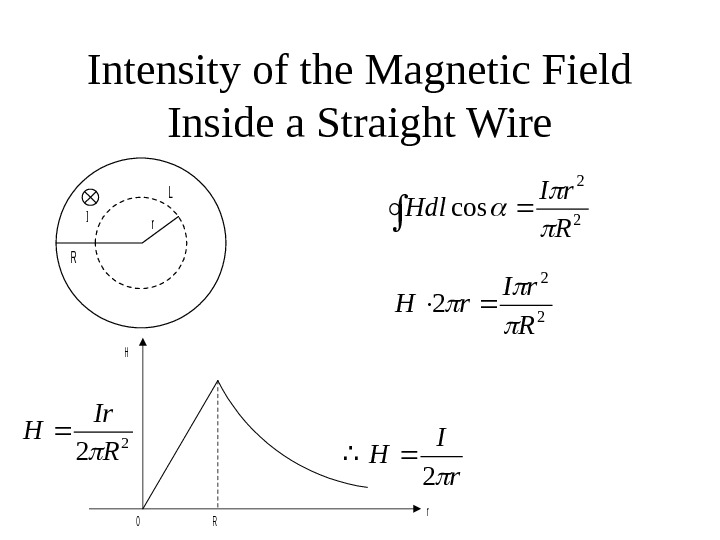Intensity of the Magnetic Field Inside a Straight Wire. L R r. I 2 2 cos R r. I Hdl 2 2 2 R r. I r. H 2 2 RIr H r R 0 H r I H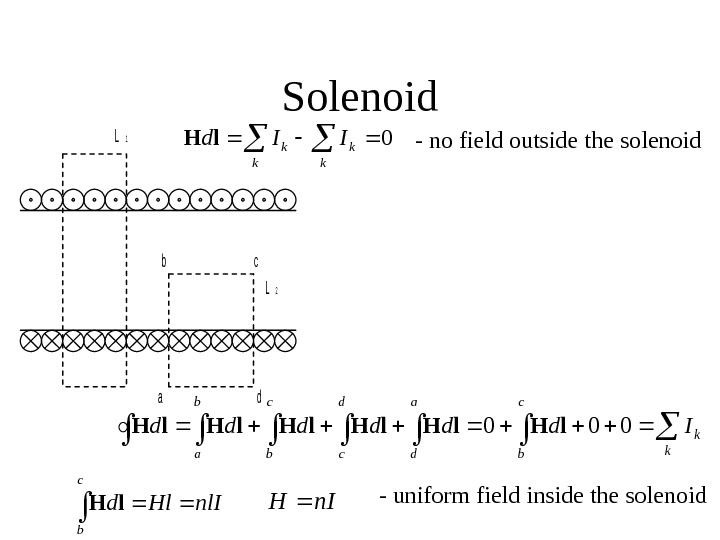Solenoid. L 1 bc L 2 ad 0 kk kk. IIdl. H c bk k d c a d b a Idddddd 000 l. Hl. Hl. H c b nl. IHldl. Hn. IH — uniform field inside the solenoid — no field outside the solenoid